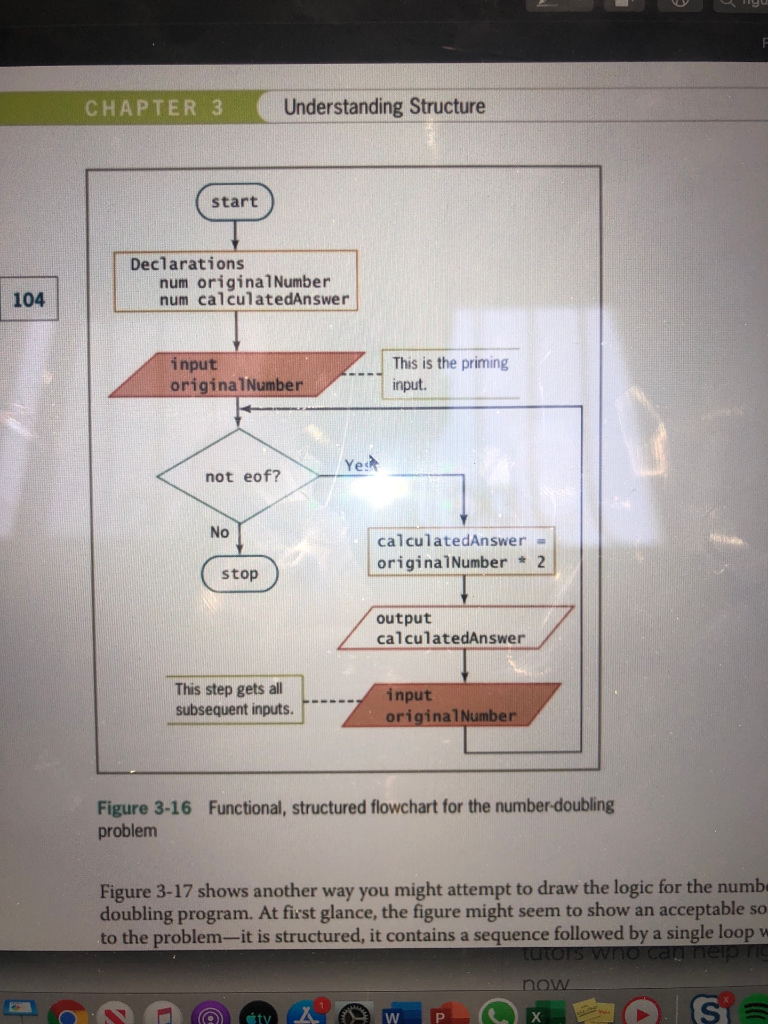# Solved: Prepare Pseudocode For A Program That Will Let A User Enter Numbers Until The Special Number 222 Is Entered (you Should Use An Indefinite Loop For This.) Once The User Entered The Special Valu

By |

Prepare pseudocode for a program that will let a user enter numbers until the special number 222 is entered (you should use an indefinite loop for this.) Once the user entered the special value, your program should output the number of numbers entered AND the sum of those numbers. You'll need a counter and an accumulator for this (see pp. 179-181 and 205-208). Make sure your loop is structured (see priming input in figure 3-16). Don't forget to comment your code. Make sure you PROMPT the user whenever requesting input from the user. Use a NAMED CONSTANT for the constant in this problem (no magic numbers)Understanding Structure CHAPTER 3 start Declarations num original Number num calculatedAnswer 104 This is the priming input originalNumber input. Yes not eof? No calculatedAnswer originalNumber 2 stop output calculatedAnswer This step gets all subsequent inputs. input originalNumber Figure 3-16 problem Functional, structured flowchart for the number-doubling Figure 3-17 shows another way you might attempt to draw the logic for the numb doubling program. At first glance, the figure might seem to show an acceptable to the problem-it is structured, it contains a sequence followed by a single loop w tutors wo can nep ri SC now